Question

The statements in the tables below are about two different chemical equilibria. The symbols have their usual meaning, for example stands for the standard Gibbs free energy of reaction and stands for the equilibrium constant. In each table, there may be one statement that is false because it contradicts the other three statements. If you find a false statement, check the box next to it. Otherwise, check the "no false statements" box under the table.

 statement false? lnK=0 ? ?G=0 ? K=1 ? ?H=T?S ?

no false statements:?

 statement false? lnK<0 ? ?H>T?S ? ?G>0 ? K<1 ?

no false statements:?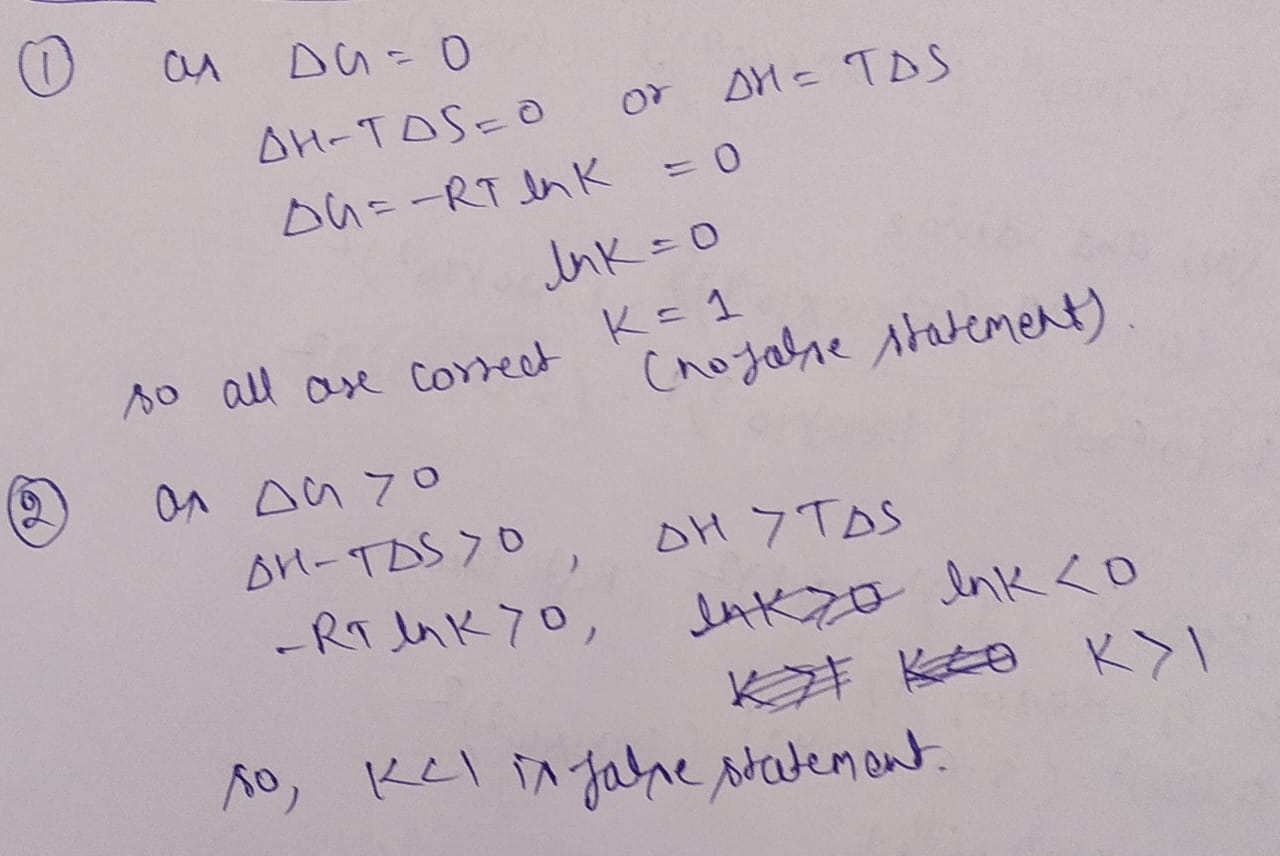If you have any questions please comment

If you satisfied with the solution please rate it thanks....

#### Earn Coins

Coins can be redeemed for fabulous gifts.

Similar Homework Help Questions
• ### The statements in the tables below are about two different chemical equilibria. The symbols have their...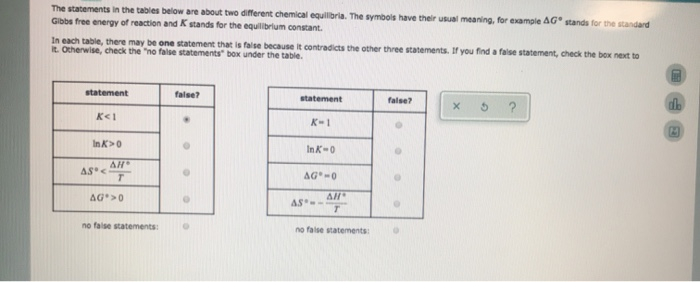The statements in the tables below are about two different chemical equilibria. The symbols have their usual meaning, for example AG stands for the standard Gibbs free energy of reaction and K stands for the equilibrium constant. In each table, there may be one statement that is faise because it contradicts the other three statements. If you find a faise statement, check the bex next to It. Otherwise, check the "no false statements" box under the table statement false? statement...

• ### The statements in the tables below are about two different chemical equilibria. The symbols have their...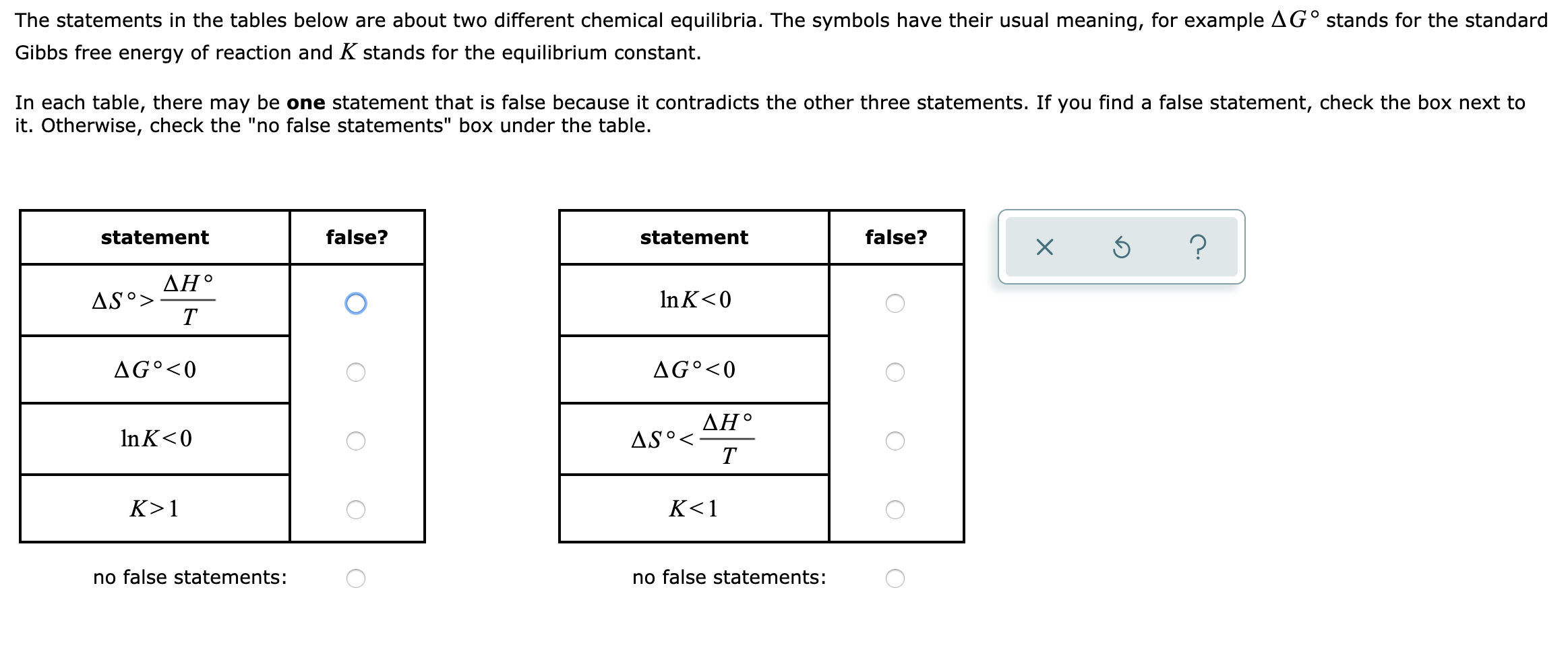The statements in the tables below are about two different chemical equilibria. The symbols have their usual meaning, for example AG° stands for the standard Gibbs free energy of reaction and K stands for the equilibrium constant. In each table, there may be one statement that is false because it contradicts the other three statements. If you find a false statement, check the box next to it. Otherwise, check the "no false statements" box under the table. statement false? statement...

• ### The statements in the tables below are about two different chemical equilibria. The symbols have their...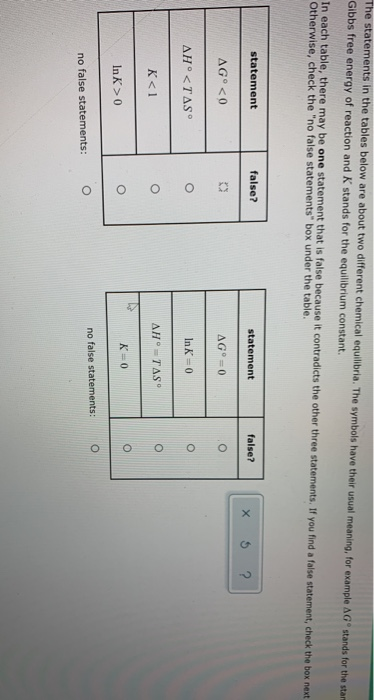The statements in the tables below are about two different chemical equilibria. The symbols have their usual meaning, for example AG stands for the stal Gibbs free energy of reaction and K stands for the equilibrium constant. In each table, there may be one statement that is false because it contradicts the other three statements. If you find a false statement, check the box next Otherwise, check the "no false statements" box under the table. statement false? statement false? X...

• ### The statements in the tables below are about two different chemical equilibria. The symbols have their...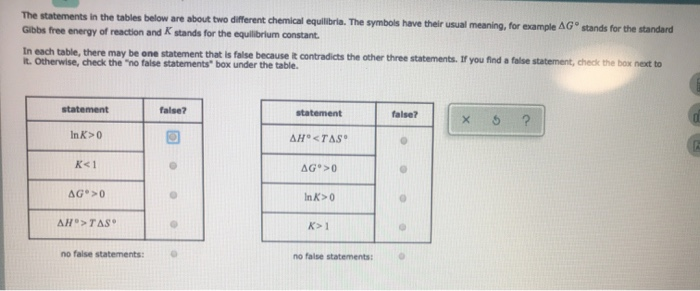The statements in the tables below are about two different chemical equilibria. The symbols have their usual meaning, for example AG Gibbs free energy of reaction and stands for the equilibrium constant. stands for the standard In each table, there may be one statement that is false because it contradicts the other three statements. If you find a false statement, check the box next to It. Otherwise, check the "no false statements box under the table. statement false? statement false?...

• ### The statements in the tables below are about two different chemical equilibria. The symbols have their...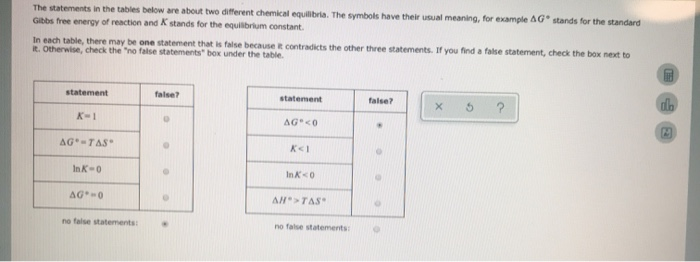The statements in the tables below are about two different chemical equilibria. The symbols have their al meaning, for example AG stands for the standard Gibbs free energy of reaction and stands for the equilibrium constant. In each table, there may be one statement that is false because it contradicts the other three statements. If you find a false statement, check the box next to it. Otherwise, check the "no false statements" box under the table statement false? statement false?...

• ### Fill in the missing chemical formulae in the tables below. acid conjugate base base conjugate acid...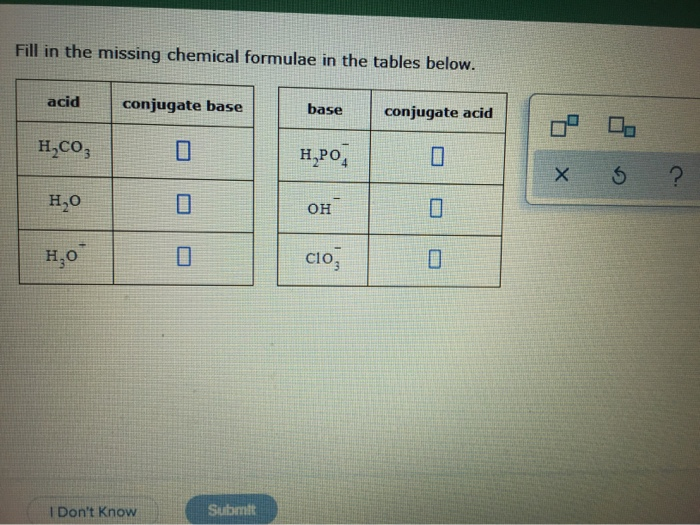Fill in the missing chemical formulae in the tables below. acid conjugate base base conjugate acid H,CO, H.PO, XS ? H,0 он H,0 clo, Submit I Don't Know At a certain temperature, the equilibrium constant K for the following reaction is 8.0 x 10 NO3(8) + NO(g) – 2 NO,(8) Use this information to complete the following table. A There will be very little NO, and NO. Suppose a 29. L reaction vessel is filled with 2.0 mol of NO3...

• ### Part 1.) Choose all of the statements from below which correctly describe issues pertaining to the...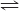Part 1.) Choose all of the statements from below which correctly describe issues pertaining to the relationship between the emf of a reaction and its Gibbs free energy change. 1. The Gibbs free energy change and emf values of a reaction have opposite algebraic signs (+ vs. -). 2. A mole of particles is more particles than a coulomb of particles. 3. A spontaneous reaction has a positive Gibbs free energy change and a negative emf. 4. A Gibbs free...

• ### A chemical engineer is studying the two reactions shown in the table below. ta constant temperature of 33.0 °C and cons...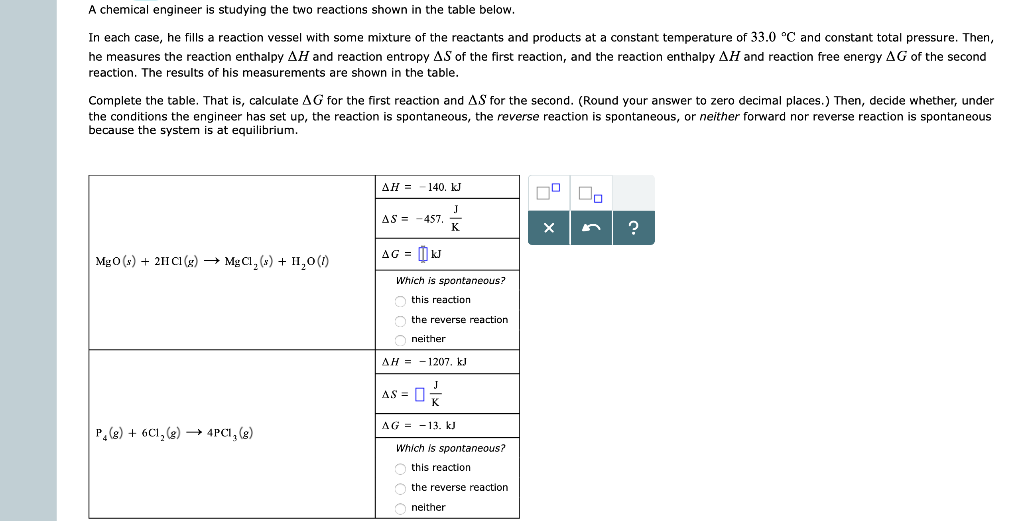A chemical engineer is studying the two reactions shown in the table below. ta constant temperature of 33.0 °C and constant total pressure. Then, In each case, he fills a reaction vessel with some mixture of the reactants and products he measures the reaction enthalpy AH and reaction entropy AS of the first reaction, and the reaction enthalpy AH and reaction free energy AG of the second reaction. The results of his measurements are shown in the table. Complete the...

• ### T=20 degrees Celsius, T= 273.13+20=293.13 Delta G= Delta H - T×Delta S. 1.What is delta S Using the tables i have provided? 2. What is the significance of the sign Delta S? *PLEASE SHOW ALL CALCULATIO...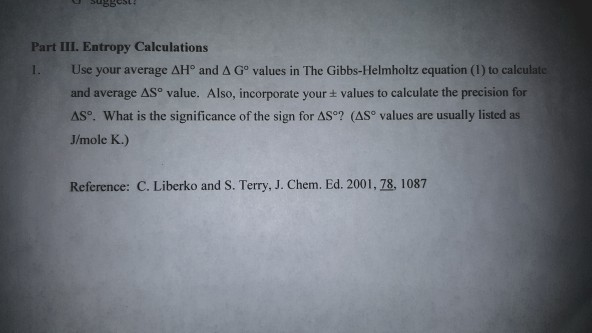T=20 degrees Celsius, T= 273.13+20=293.13 Delta G= Delta H - T×Delta S. 1.What is delta S Using the tables i have provided? 2. What is the significance of the sign Delta S? *PLEASE SHOW ALL CALCULATIONS* uBBOsty Part III. Entropy Calculations 1. Use your average AHo and A Go values in The Gibbs-Helmholtz equation (1) to calculate and average ASo value. Also, incorporate your ± values to calculate the precision for Δ. What is the significance of the sign for...

• ### A chemical engineer is studying the two reactions shown in the table below. In each case,...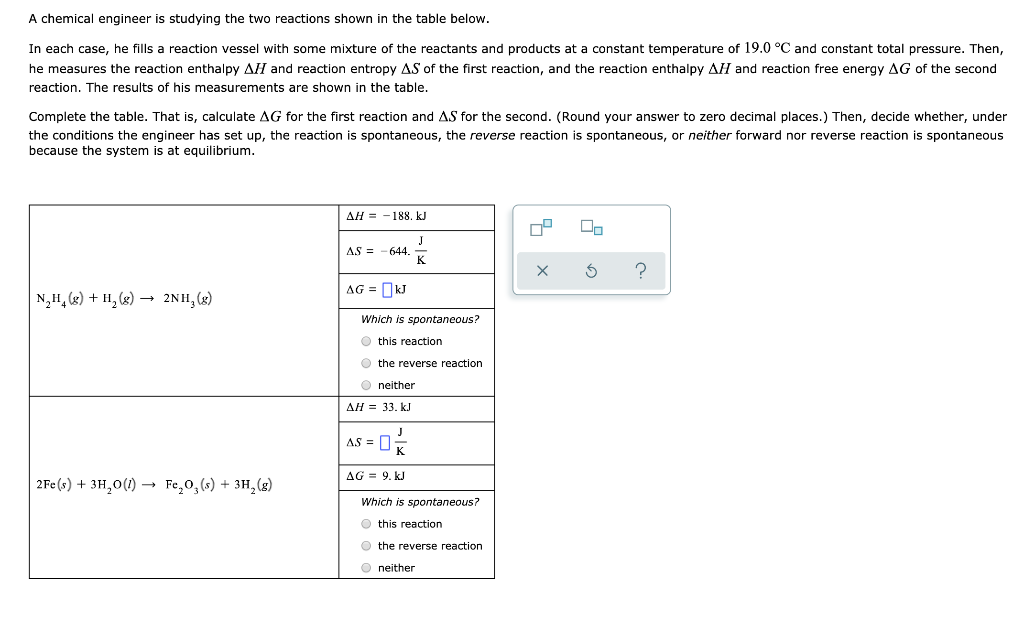A chemical engineer is studying the two reactions shown in the table below. In each case, he fills a reaction vessel with some mixture of the reactants and products at a constant temperature of 19.0°C and constant total pressure. Then, he measures the reaction enthalpy AH and reaction entropy AS of the first reaction, and the reaction enthalpy AH and reaction free energy AG of the second reaction. The results of his measurements are shown in the table. Complete the...